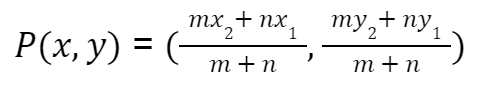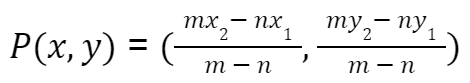Maths
Study Material

# Section Formula | Internal and External Division |

Section formula is basically used to find the coordinates of the point that divides the line segment into any ratio. Read more about the section formula and its examples in the following section.

4 minutes longHesap Oluştur

Got stuck on homework? Get your step-by-step solutions from real tutors in minutes! 24/7. Unlimited.

Section formula is basically used to find the coordinates of the point that divides the line segment (externally or internally) into any ratios. The section formula is very helpful in maths as well as in physics. In maths, it is used to find incenters, centroids or excenters of a triangle. In physics, it is used to find the center of equilibrium points, mass, etc.

## Section Formula Definition

The formula which is used to determine the coordinates of a point that divides the line segment joining two points into two parts with the given ratio is known as the section formula. Also, a special case of this is the midpoint of a line segment, which divides the line segment in two equal parts in the ratio 1:1.

#### Section formula is mainly divided into two parts, which are:

• Internal section formula
• External section formula

## Internal Section Formula

It is the Formula for Internal Division. When a line segment is divided internally in the ratio m:n, we use the Internal section formula. That is when the point P lies anywhere between the points A and B.
So, the coordinates of the point P(x,y) which divides the line segment joining the points A(x1,y1) and B(x2,y2) internally in the ratio m:n is• x, and y are the coordinates of point P.
• (x₁, y₁) are the Cartesian coordinates of point A.
• (x₂, y₂) are the Cartesian coordinates of point B.
• m and n are the ratio values in which P divides the line internally.

## External section formula

When the point P lies on the external part of the line segment, the section formula for the external division is used for its coordinates. A point on the external part of the segment means when you extend the segment than its actual length the point lies there.
Also, the coordinates of the point P(x,y) which divides the line segment joining the points A(x₁, y₁) and B(x₂, y₂) externally in the ratio m:n is• x, and y are the coordinates of point P.
• (x₁, y₁) are the Cartesian coordinates of point A.
• (x₂, y₂) are the Cartesian coordinates of point B.
• m and n are the ratio values in which P divides the line externally.

## Special case

• The midpoint of a line segment divides the line segment in the ratio 1 : 1
• The coordinates of a point P (midpoint) which divides the line segment AB in the ratio 1:1 is [(x₂+x₁)/2, (y₂+y₁)/2]
• Also called the Midpoint formula.

## Problems on Section Formula

Example 1: The coordinates of point P (x, y) where it divides the line segment joining (7, – 6) and (3, 4) in the ratio 1 : 2 internally lies in which quadrant ?

Solution: Given coordinates are A (7, -6) and B (3, 4)

Let P (x, y) be a point which divides the line segment in the ratio of 1 : 2 i.e m : n = 1 : 2

Now using the formula P(x, y) = {(m×x₂ + n×x₁)/(m+n), (m×y₂ + n×y₁)/(m+n)} as P is dividing internally.

=> P(x, y) = {(1×3 + 2×7 ) / (1+2), (1×4 + 2×(-6))/(1+2)}

=> P(x, y) = (17/3, -8/3)

P(x, y) = (17/3, -8/3)

Example 2: Find the coordinate of the point P which divides the points A (−3, −1), B (−1, 0) in a ratio of 2:3 externally, with the help of section formula.

Solution: Given, (x₁, y₁) is (-3, −1) and (x₂, y₂) is (-1, 0) also m : n = 2:3
To find the coordinates of the point P(x, y)

Using the section formula for external division

P(x, y) = {(m×x₂ + n×x₁)/(m+n), (m×y₂ + n×y₁)/(m+n)}

=> P(x, y) = ((2×(-1) – 3×(-3))/(2-3), (2×(0) – 3×(-1))/2-3)

=> P(x, y) = ((-2+9)/(-1), 3/(-1))

P(x,y) = (-7, -3)

Answer: Hence, The coordinates of point P are (-7, -3).

##### More Examples

Example 3: By using the section formula, find out coordinates of the center of a circle if the endpoints of the diameter of the circle are (3, -4) and (-7, 4) respectively.

Solution: To find the coordinates of the center of a circle, say C(x, y).

Given: Coordinates of the endpoint of the diameter: (3, -4) and (-7, 4)

Using the midpoint formula since the center of the circle lies at the exact center of the diameter of the circle.

C(x, y) = [(x₂+x₁)/2, (y₂+y₁)/2]
Putting the respective values, we get

C(x, y) = (((-7)+ 3 )/2, (4+ (-4))/2))

C(x, y) = (-2, 0)

Answer: Hence, The coordinates of the center of the circle are (-2, 0).

## Picked For You

Furthermore, these are some topics which might interest you: